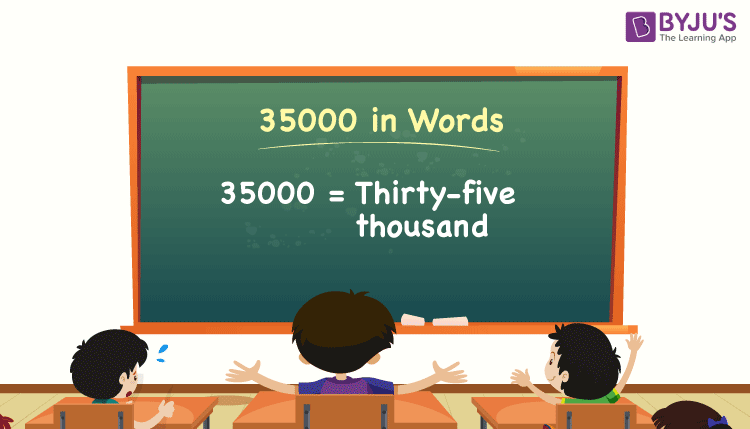# 35000 in Words

We can write 35000 in words as Thirty-five Thousand. Suppose a book has 35000 words of content in it; we can say that this book contains thirty-five thousand words. We can derive the conversion of 35000 to words with the help of a place value table. Also, note that 35000 is a cardinal number since it represents a specific quantity.

 35000 in words: Thirty-five thousand. Thirty-five thousand in numerical form: 35000.

35000 in English words

We generally write numbers in words using the English alphabet. Thus, we can read 35000 in English as “Thirty-five Thousand”.## How to Write 35000 in Words?

We can convert 35000 to words using a place value chart. Let’s look at the conversion of the number 35000 to words.

The number 35000 has five digits, so let’s make a chart that shows the place value up to 5 digits.

 Ten thousand Thousands Hundreds Tens Ones 3 5 0 0 0

Thus, we can write the expanded form as:

3 × Ten thousand + 5 × Thousand + 0 × Hundred + 0 × Ten + 0 × One

= 3 × 10000 + 5 × 1000 + 0 × 100 + 0 × 10 + 0 × 1

= 30000 + 5000

= 35000

= Thirty-five thousand

Therefore, 35000 in words is written as Thirty-five thousand.

Interesting way of writing 35000 in words:

3 = Three

35 = Thirty-five

350 = Three hundred and fifty

3500 = Three thousand five hundred

35000 = Thirty-five thousand

### Facts About the Number 35000

35000 is a natural number that is the successor of 34999 and the predecessor of 35001.

35000 in words – Thirty-five thousand

Is 35000 an odd number? – No

Is 35000 an even number? – Yes

Is 35000 a perfect square number? – No

Is 35000 a perfect cube number? – No

Is 35000 a prime number? – No

Is 35000 a composite number? – Yes

Get the conversion of some numbers to words

435000 in words = Four lakh thirty-five thousand

3500 rupees in words = Three thousand five hundred

135000 in words = One lakh thirty-five thousand

235000 in words = Two lakh thirty-five thousand

Rs. 5,35,000 in words = Five lakh thirty-five thousand rupees

## Frequently Asked Questions on 35000 in Words

Q1

### How do you write Rs. 35000 in words?

We can write Rs. 35000 in words as Thirty-five thousand rupees.

Q2

### How do you say 35000 in English?

We can express 35000 in words as Thirty-five thousand. If this number represents the money or the cost of something, we say that the amount is Thirty-five thousand rupees.

Q3

### Write 35000 in words on a cheque.

On a cheque, we generally write 35000 in words as Thirty-five thousand rupees only.

Q4

### How do you write 31500 in words?

31500 can be written in words as Thirty-one thousand and five hundred.

Test your Knowledge on 35000 in Words